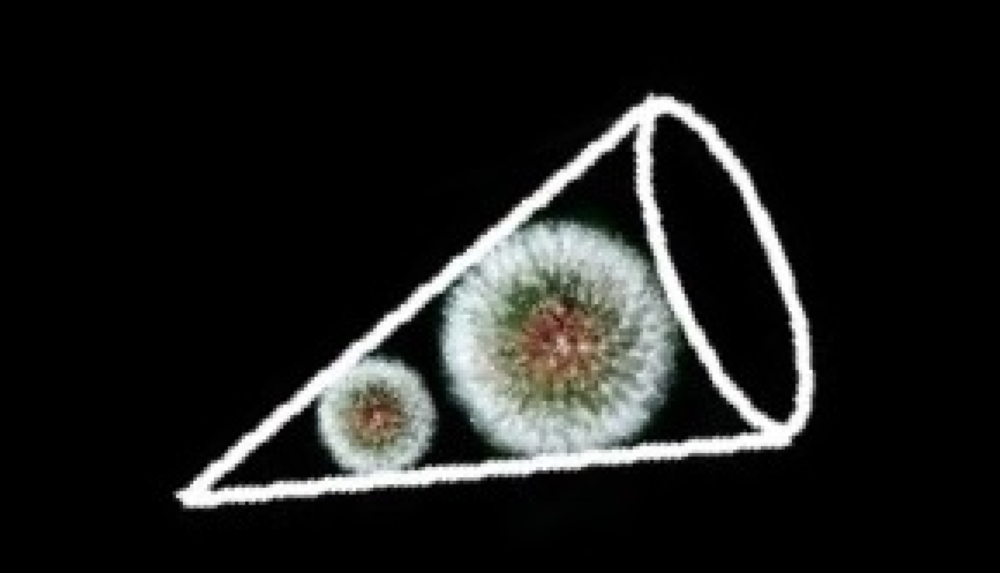# Dandelin’s Delightful Spheres

## An ingenious use of spheres sheds light on this conic section

Mathematicians have been slicing open cones since the 4th Century BC. Here, we study one of these famous cross sections — the ellipse. Along the way, we’ll discover a beautiful proof from Germinal Dandelin of the 19th Century.

Slice a right circular cone at an angle to the horizontal. The cross-section forms an ellipse.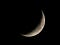A fingernail moon: inspiration for poets and mathematicians alike (Source)

# The Area of a Lune

## Another No-Algebra Geometry Puzzle

This one comes from Michael Penn, who in turn got it from a dead mathematician with a beard. You can check out Penn’s solution on his YouTube channel. The solution below is my own.

Figure 1 shows a right triangle parachuting to the ground with a bedsheet.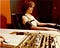Woman with 70’s hairstyle demonstrates polygraph. (Source: FBI. Really.)

# A Bayes’ Theorem Lie Detector

## Your help is requested

Dear Readers,

Here is a neat application of Bayes’ Theorem. Imagine that a professor gives her students an exam. She wants to know how likely it is than any of them cheated. But she can’t just ask them. Can she even expect students to be report honestly on an anonymous survey? (Is it really anonymous? Really?)

I recently posted this on Reddit:

`I am working on an article on Bayes Theorem and statistical significance, and I am asking for your help, dear Redditors! Here’s what I need you to do:1. Flip a coin.2. If the coin turns up…`

# A General Binomial Theorem

## How to deal with negative and fractional exponents

The Binomial Theorem is commonly stated in a way that works well for positive integer exponents.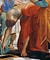Actual image of Euclid solving a problem he found on Instagram. (Source.)

# The Circle-Parabola Problem

## No Calculus. No Algebra. Just Plane Geometry.

Last week, Dr. Peyam (of YouTube fame) published a video of a geometry problem he found on Instagram. The solution, he says, “uses a lot of beautiful geometry and even more beautiful calculus.” (It does indeed. Check it out.)

I am going to attack the same problem using methods that predate calculus — and even algebra. Here goes!

A parabola formed by the graph of y=x² cradles a circle of radius 1. The circle touches the parabola at exactly two points. What are the coordinates of the centre of the circle?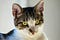Actual image of cat learning Mamikon’s Theorem (Source)

# The 2 Washers Puzzle

## A surprising geometry lies behind the solution.

You have two circular washers. Each is made of the same material; each has the same thickness. The central holes of the two washers have different diameters.

On each washer, a straight line is drawn from one edge to the other, so it just touches the central hole. The two straight lines each have the same length (Figure 1).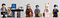How to choose from so many wonderful candidates? Use math! (Source)

# Knowing When to Quit — An Optimal Stopping Problem

## Using mathematics to maximize results

The Secretary Problem is classic Decision Theory scenario. You must choose one of N possible candidates. You have an objective way of ranking them. The proviso is that after you examine a candidate, you must either choose or reject that candidate. There is go going back for a second look.

For example, in Princess Alice and the 1,001 Suitors, once Alice met a potential husband, she either decapitated him or married him.

`What strategy will maximize the probability of choosing the best candidate?`

Suppose you are at an automobile auction. Each of 19 cars will be displayed, one at a…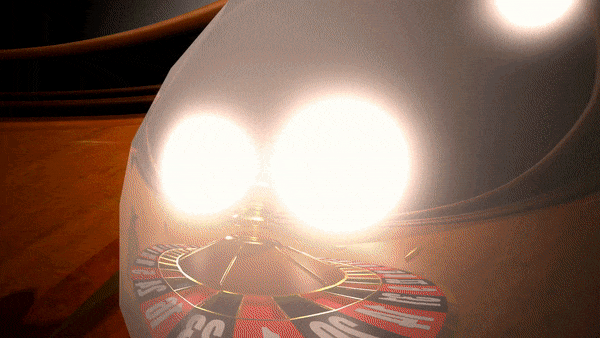I couldn’t find a graphic of ininitely sided dice. So sue me. (Source)

# This Math Puzzle Looks Hard

## Infinitely-sided dice produce a surprising result.

Here’s the challenge. You have three infinitely-sided dice. When you roll one of these dice, you get a Real Number between 0 and 1. So when you roll three of these dice, you get a Real Number between 0 and 3. Capish?

Here’s the question. You roll the three dice. You square the outcome of each die.

`What are the odds that the sum of the three squares will be less than or equal to 1?`

These examples should clarify:

# Solution to that Find the Zeros Puzzle

## Some Golden Triangle Trigonometry.😎

Here’s hoping you all had fun with last week’s Find the Zeros puzzle. As promised, here is my solution.

(Livestream recording on YouTube is here.)

You may recall, we are looking for solutions to this function between 0 and :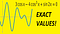Can you find the six roots between 0 and 2π?

# A Math Puzzle and a Free Book

## No calculus required!

Your mission — should you choose to accept it — is to find the six roots between 0 and of this function: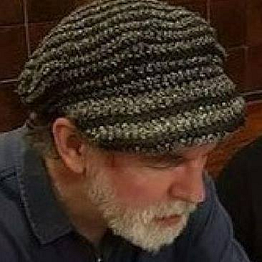## Adam Hrankowski

I am a maths/physics tutor in BC. You can find my stuff on YouTube: youtube.com/c/mathadam My Virtual Tip Jar: https://ko-fi.com/mathadam Thanks!